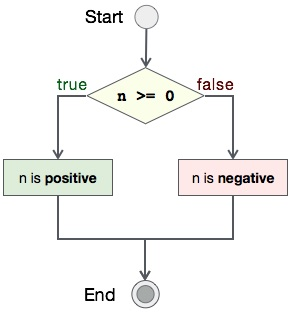# Find Positive-Negative Program In C

Like finding a number is even or odd, finding a number positive or negative is also very simple program to write. We shall learn the use of conditional statement `if-else` in C.

## Algorithm

Algorithm of this program is very easy −

```START
Step 1 → Take integer variable A
Step 2 → Assign value to the variable
Step 3 → Check if A is greater than or equal to 0
Step 4 → If true print A is positive
Step 5 → If false print A is negative
STOP
```

## Flow Diagram

We can draw a flow diagram for this program as given below −## Pseudocode

```procedure positive_negative()

IF ( number ≥ 0 )
PRINT number is positive
ELSE
PRINT number is negative
END IF

end procedure
```

## Implementation

Implementation of this algorithm is given below −

```#include <stdio.h>

int main() {
int number = -2;

if (number >= 0)
printf("%d is positive\n", number);
else
printf("%d is negative\n", number);

return 0;
}
```

## Output

Output of the program should be −

```-2 is negative
```
simple_programs_in_c.htm